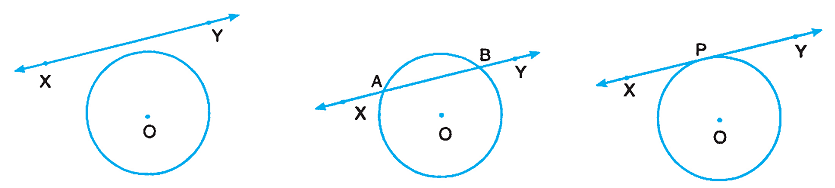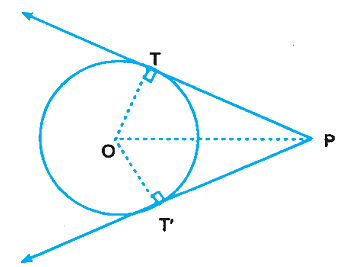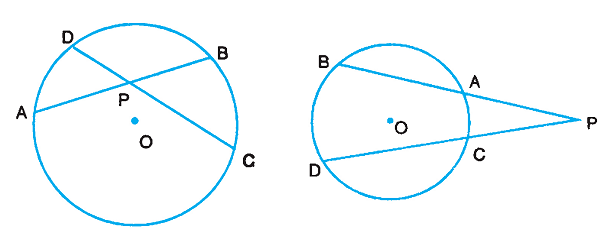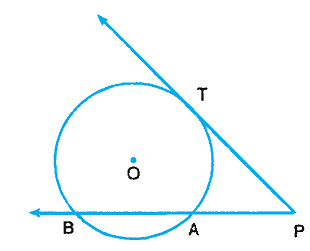# Secants and Tangents

In case of intersection of a line and a circle, the following three possibilities are there:

1. The line does not intersect the circle at all, i.e., the line lies in the exterior of the circle.
2. The line intersects the circle at two distinct points. In that case, a part of the line lies in the interior of the circle, the two points of intersection lie on the circle and the remaining portion of the line lies in the exterior of the circle.
3. The line touches the circle in exactly one point.Tangent

A line which touches a circle at exactly one point is called a tangent line and the point where it touches the circle is called the point of contact.

Secant

A line which intersects the circle in two distinct points is called a secant.

A tangent is the limiting position of a secant when the two points of intersection coincide.

### Properties

A radius, though the point of contact of tangent to a circle, is perpendicular to the tangent at that point.

Tangent From Point Outside Circle

From an external point, two tangents can be drawn to a circle. The lengths of two tangents from an external point are equal.

PT = PT′

The tangents drawn from an external point to a circle are equally inclined to the line joining the point to the centre of the circle.

∠OPT = ∠OPT′Intersecting Chords

If two chords AB and CD of a circle intersect at a point P (inside or outside the circle), then

PA × PB = PC × PDIntersecting Secant and Tangent

If PAB is a secant to a circle intersecting the circle at A and B, and PT is a tangent to the circle at T, then

PA × PB = PT2Angles Made by Tangent & Chord

The angles formed in the alternate segments by a chord through the point of contact of a tangent to a circle is equal to the angle between the chord and the tangent. This result is more commonly called as Angles in the Alternate Segment.

∠PRQ = ∠QPY

∠QPX = ∠QSPIf a line makes with a chord angles which are equal respectively to the angles formed by the chord in alternate segments, then the line is a tangent to the circle.